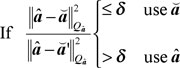# Integer ambiguity validation: Still an open problem?

July 30, 2007  -By Sandra Verhagen

High-precision Global Navigation Satellite System (GNSS) positioning results are obtained with carrier phase measurements, once the integer cycle ambiguities have been successfully resolved.

The position solution is obtained in four steps:

1. Float solution:least-squares, discarding integer nature.

2. Integer solution: real-valued float ambiguities mapped to integer-valued ambiguities.Examples of integer estimators (Teunissen, 1998a):

• Integer Least-Squares: optimal, requires search to obtain solution.
• Integer Bootstrapping: may perform close to optimal (decorrelating ambiguity transformation required), no search required (e.g. widelaning, CIR, TCAR).
• Integer Rounding: the simplest of all methods.

3. Integer acceptance test: decision whether or not to accept integer ambiguity solution. Examples: ratio test, distance test, projector test.

4. Fixed solution: if the integer solution is accepted, the fixed baseline is computed.

The third step is often referred to as the ‘integer validation’ problem. In Verhagen (2004) this problem was addressed, and different approaches were compared.

As an example, we will now consider the popular ratio test, which is defined as:Where ȃ is the float solution with Qȃ, the corresponding variance matrix; and ă and ă’, the corresponding integer estimate and the second-best integer candidate, respectively; δ is the critical value. Note: in practice, often the reciprocal of the ratio test, as specified here, is used.

The underlying principle of the ratio test can be explained with a 2-dimensional example, see the figure below. Assume we have two ambiguities in our model. The black hexagons are the so-called integer least-squares pull-in regions: if the float ambiguity estimate falls inside a certain hexagon, the integer solution is equal to the grid point in the center of this pull-in region. Applying the ratio test, however, implies that this integer solution is only accepted if it falls inside one of the red regions. Otherwise, the float ambiguity is considered to be too close to the boundary of a pull-in region, such that the integer solution is not sufficiently more likely than the second-best integer candidate.Note that the size of the regions is controlled by the critical value, δ, see Verhagen and Teunissen (2006), and Teunissen and Verhagen (2007), where it is described how this value should be chosen.

It can be seen that the acceptance regions are invariant for translations with an integer value. As such, the ratio test is invariant to integer biases. In fact, the ratio test is not suitable for testing the correctness of the solution. A model error, such as a bias in the observations, will propagate into the float ambiguities, but it does not necessarily mean that the float ambiguity will be close to the boundary of a pull-in region.

Hence, the ratio test is not a model validation test, and should only be applied in order to test whether or not the integer solution can be regarded sufficiently more likely than any other integer candidate.

With regard to GNSS model validation, we can make the following remarks:

1. Classical testing theory based on statistical hypothesis testing is not applicable due to the integer nature of the carrier-phase ambiguities (Teunissen, 1998b).

2. Testing theory for testing the presence/absence of a model error is not yet available.

3. Questions that need to be answered are:

• What are the appropriate test statistics?• How are they distributed under the null-hypothesis and alternative hypothesis?
• What are the appropriate acceptance/rejection regions?

References

Teunissen, P.J.G. (1998). “A class of unbiased integer GPS ambiguity estimators.” Artificial Satellites, 33(1): 4-10.

Teunissen, P.J.G. (1998b). “GPS carrier phase ambiguity fixing concepts.” In: Teunissen, P.J.G. and A Kleusberg. GPS for Geodesy, Springer-Verlag, Berlin.

Teunissen, P.J.G. and Verhagen, S. (2007). “GNSS phase ambiguity validation: a review.” Proceedings Space, Aeronautical and Navigational Electronics Symposium SANE2007, The Institute of Electronics, Information and Communication Engineers (IEICE), Japan, 107(2): 1-6.

Verhagen, S. (2004). “Integer ambiguity validation: an open problem?” GPS Solutions, 8(1): 36-43.

Verhagen, S. and Teunissen, P.J.G. (2006). “New global navigation satellite system ambiguity resolution method compared to existing approaches.” Journal of Guidance, Control and Dynamics, 29(4): 981-991.

Dr.ir. Sandra Verhagen,
DEOS-MGP, TU Delft